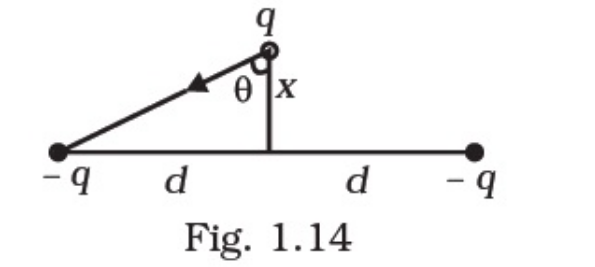Deepak Scored 45->99%ile with Bounce Back Crack Course. You can do it too!

# Two charges –q each are fixed separated by distance 2d.

Question:

Two charges –each are fixed separated by distance 2d. A third charge q of mass m placed at the mid-point is displaced slightly by x (x<<d) perpendicular to the line joining the two fixed charged as shown in Fig. 1.14.Show that q will perform simple harmonic oscillation of time period.

$T=\left[\frac{8 \pi^{3} \varepsilon_{0} m d^{3}}{q^{2}}\right]^{1 / 2}$

Solution:

Net force F on q towards the centre $\mathrm{O}$

$F=2 \frac{q^{2}}{4 \pi \varepsilon_{\theta} r^{2}} \cos \theta=-\frac{2 q^{2}}{4 \pi \varepsilon_{\theta} r^{2}} \cdot \frac{x}{r}$

$F=-\frac{2 q^{2}}{4 \pi \varepsilon_{0}} \cdot \frac{x}{\left(d^{2}+x^{2}\right)^{1 / 2}}$

$\approx-\frac{2 q^{2}}{4 \pi \varepsilon_{0} d^{3}} x=-k ;$ for $x< Thus, the force on the third charge$q$is proportional to the displacement and is towards the centre of the two other charges. Therefore, the motion of the third charge is harmonic with frequency$\omega=\sqrt{\frac{K}{m}}\Rightarrow T=\frac{2 \pi}{\omega}=2 \pi \sqrt{\frac{m}{K}}\Rightarrow T=2 \pi \sqrt{\frac{m .4 \pi e_{\nu} d^{3}}{2 q^{2}}}=\left[\frac{8 \pi^{3} \varepsilon_{0} m d^{3}}{q^{2}}\right]^{1 / 2}\$